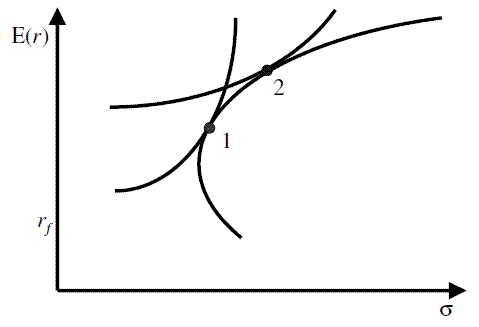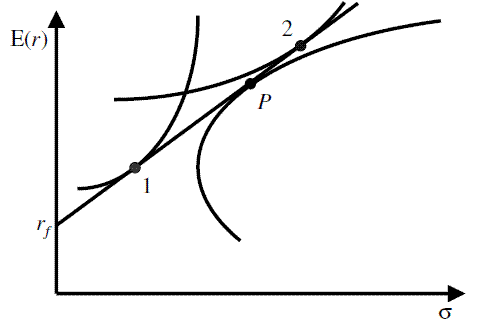### Why should I choose AnalystNotes?

Simply put: AnalystNotes offers the best value and the best product available to help you pass your exams.

##### Subject 7. Optimal Portfolio

The efficient frontier only considers the investments in risky assets. However, investors may choose to invest in a risk-free asset, which is assumed to have an expected return commensurate with an asset that has no standard deviation (i.e., zero variance) around the expected return. That is, a risk-free asset's expected return is entirely certain; it is known as the risk-free rate of return (RFR). Therefore, a risk-free asset lies on the vertical axis of a portfolio graph.

When a risk-free asset is combined with a risky portfolio, a graph of possible portfolio risks-return combinations becomes a straight line between the two assets.

Assume the proportion of the portfolio the investor places in the tangency portfolio P is wP:

• The expected rate of return for the new portfolio is the weighted average of the two returns: E(R) = (1 - wP) Rf + wP E(RT)
• The standard deviation of the new portfolio is the linear proportion of the standard deviation of the risky asset portfolio P: σportfolio = wP σP

The introduction of a risk-free asset changes the efficient frontier into a straight line. This straight efficient frontier line is called the Capital Market Line (CML) for all investors and the Capital Allocation Line (CAL) for one investor.

• Investors at point rf have 100% of their funds invested in the risk-free asset.
• Investors at point P have 100% of their funds invested in portfolio P.
• Between rf and P, investors hold both the risk-free asset and portfolio P. This means investors are lending some of their funds (buying the risk-free asset).
• To the right of P, investors hold more than 100% of portfolio P. This means they are borrowing funds to buy more of portfolio P. This represents a levered position.

Investors will choose the highest CAL (i.e., the CAL tangent to the efficient frontier). This portfolio is the solution to the optimization problem of maximizing the slope of the CAL.Now, the line rf-P dominates all portfolios on the original efficient frontier. Thus, this straight line becomes the new efficient frontier.

Separation Theorem

Investors make different financing decisions based on their risk preferences. The separation of the investment decision from the financing decision is called the separation theorem. The portfolio choice problem can be broken down into two tasks:

• Choosing P, a technical matter (can be done by the broker)
• Deciding on the proportion to be invested in P and in the riskless asset.

Optimal Investor Portfolio

We can combine the efficient frontier and/or capital allocation line with indifference curves. The optimal portfolio is the portfolio that gives the investor the greatest possible utility.

• Two investors will select the same portfolio from the efficient set only if their utility curves are identical.
• Utility curves to the right represent less risk-averse investors; utility curves to the left represent more risk-averse investors.

This is portfolio selection without a risk-free asset:The optimal portfolio for each investor is the highest indifference curve that is tangent to the efficient frontier.

This is portfolio selection with a risk-free asset:The optimal portfolio for each investor is the highest indifference curve that is tangent to the capital allocation line.